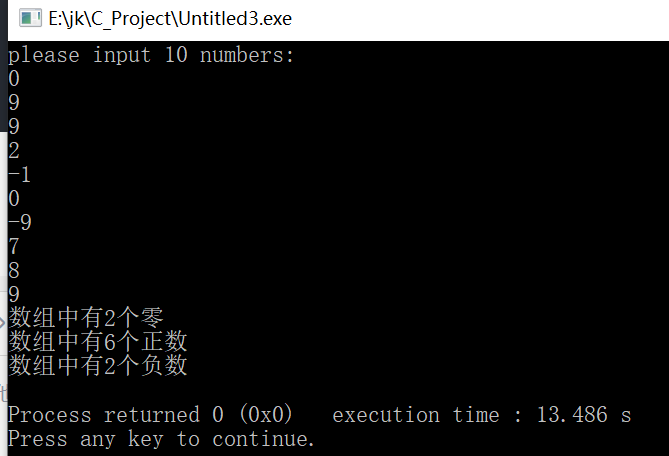2021-07-23 12:02

# 编程统计数组a中的正数、0、负数的个数(20)

• 好问题 提建议
• 收藏

#### 2条回答默认 最新

•奋斗的小小鱼 2021-07-23 14:52
已采纳
``````
#include<iostream>
using namespace std;

int main()
{
int x=0,y=0,z=0;
int a;
cout<<"请输入10个数字：:"<<endl;
for(int i=0;i<10;i++)
{
cin>>a[i];
}

for(int j=0;j<10;j++)
{
if(a[j]==0) x++;

else if(a[j]>0) y++;

else z++;

}

cout<<"数组中有"<<x<<"个零"<<endl;
cout<<"数组中有"<<y<<"个正数"<<endl;
cout<<"数组中有"<<z<<"个负数"<<endl;

return 0;
}

``````已采纳该答案
评论
解决 无用
打赏 举报
•qzjhjxj 2021-07-23 12:23

这题是送分题：

``````#include <stdio.h>
#define N 20

int main()
{
int a[N]={0,1,7,5,-8,2,-9,25,0,56,-12,-75,29,4,-9,45,47,9,0,-70},
i,cnt_p=0,cnt_n=0,cnt_f=0;
for(i=0;i<N;i++){
if(a[i]>0)       cnt_p++;
else if(a[i]==0) cnt_f++;
else if(a[i]<0)  cnt_n++;
}
printf(">0:%d, =0:%d, <0:%d\n",cnt_p,cnt_f,cnt_n);
return 0;
}

``````
评论
解决 无用
打赏 举报